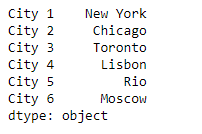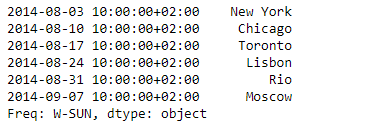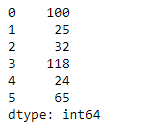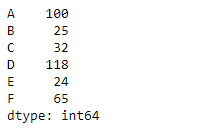Related Articles

# Python | Pandas Series.set_axis()

• Last Updated : 03 Sep, 2021

Pandas series is a One-dimensional ndarray with axis labels. The labels need not be unique but must be a hashable type. The object supports both integer- and label-based indexing and provides a host of methods for performing operations involving the index.

Pandas Series.set_axis() function is used to assign desired index to given axis. Indexes for column or row labels can be changed by assigning a list-like or Index.

Attention geek! Strengthen your foundations with the Python Programming Foundation Course and learn the basics.

To begin with, your interview preparations Enhance your Data Structures concepts with the Python DS Course. And to begin with your Machine Learning Journey, join the Machine Learning - Basic Level Course

Syntax: Series.set_axis(labels, axis=0, inplace=None)
Parameter :
labels : The values for the new index.
axis : The axis to update. The value 0 identifies the rows, and 1 identifies the columns.
inplace : Whether to return a new %(klass)s instance.
Returns : renamed : series

Example #1: Use Series.set_axis() function to reset the axis of the given Series object.

## Python3

 `# importing pandas as pd``import` `pandas as pd` `# Creating the Series``sr ``=` `pd.Series([``'New York'``, ``'Chicago'``, ``'Toronto'``, ``'Lisbon'``, ``'Rio'``, ``'Moscow'``])` `# Create the Index``index_ ``=` `[``'City 1'``, ``'City 2'``, ``'City 3'``, ``'City 4'``, ``'City 5'``, ``'City 6'``]` `# set the index``sr.index ``=` `index_` `# Print the series``print``(sr)`

Output :Now we will use Series.set_axis() function to reset the index of the given series object

## Python3

 `# Create the Index``didx ``=` `pd.DatetimeIndex(start ``=``'2014-08-01 10:00'``, freq ``=``'W'``,``                     ``periods ``=` `6``, tz ``=` `'Europe/Berlin'``)`  `# reset the index``sr.set_axis(didx, inplace ``=` `True``)` `# Print the series``print``(sr)`

Output :As we can see in the output, the Series.set_axis() function has successfully reset the index of the given Series object.

Example #2: Use Series.set_axis() function to reset the axis of the given Series object.

## Python3

 `# importing pandas as pd``import` `pandas as pd` `# Creating the Series``sr ``=` `pd.Series([``100``, ``25``, ``32``, ``118``, ``24``, ``65``])` `# Print the series``print``(sr)`

Output :Now we will use Series.set_axis() function to reset the index of the given series object

## Python3

 `# Assign the new index``sr.set_axis([``'A'``, ``'B'``, ``'C'``, ``'D'``, ``'E'``, ``'F'``], inplace ``=` `True``)` `# print the series``print``(sr)`

Output :As we can see in the output, the Series.set_axis() function has successfully reset the index of the given Series object.

My Personal Notes arrow_drop_up